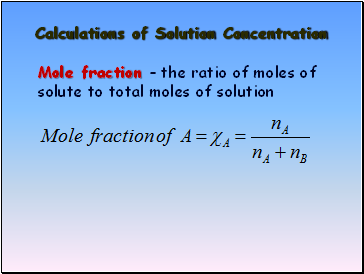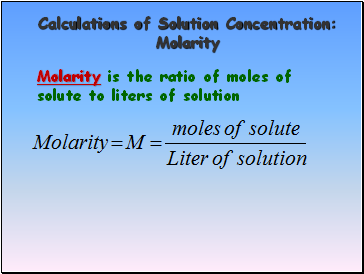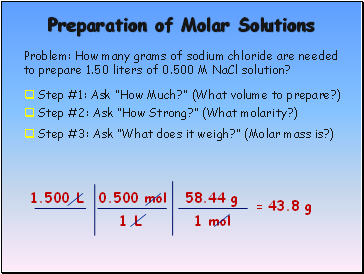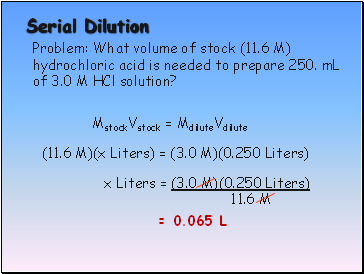# Calculation of Solution ConcentrationPage 1

#### WATCH ALL SLIDES

Slide 1Solution Concentration

Slide 2## Calculations of Solution Concentration

Mole fraction – the ratio of moles of solute to total moles of solution

Slide 3Calculations of Solution Concentration: Molarity

Molarity is the ratio of moles of solute to liters of solution

Slide 4## Preparation of Molar Solutions

Problem: How many grams of sodium chloride are needed to prepare 1.50 liters of 0.500 M NaCl solution?

Step #1: Ask “How Much?” (What volume to prepare?)

1.500 L

Step #2: Ask “How Strong?” (What molarity?)

0.500 mol

1 L

Step #3: Ask “What does it weigh?” (Molar mass is?)

58.44 g

1 mol

= 43.8 g

Slide 5## Serial Dilution

Problem: What volume of stock (11.6 M) hydrochloric acid is needed to prepare 250. mL of 3.0 M HCl solution?

MstockVstock = MdiluteVdilute

(11.6 M)(x Liters) = (3.0 M)(0.250 Liters)

x Liters = (3.0 M)(0.250 Liters)

11.6 M

= 0.065 L